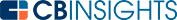# What are Financial Ratios?Financial ratios are numerical tools that compare the relationships between different figures on a company’s financial statement. There are dozens of different financial ratios that analysts and investors can use to determine the efficacy of an investment and the performance of a business.

## Why are financial ratios used?

A financial ratio is a numerical representation of a relationship between two financial figures. It gives investors a clearer picture of the value of a business. For example, knowing that a company is valued at \$10M tells us relatively little.

However, knowing that the company has a quick ratio of 1:3:1 would highlight to investors that the company’s liabilities are well covered by operating cash flow.

Below are some common financial ratios.

## Working capital ratio

The working capital ratio, also referred to as the current ratio, is a representation of liquidity. It is used to quickly determine whether a business is capable of meeting its debt paymentsIt can be calculated by dividing total current assets by total current liabilities.

A ratio between 1.2 and 2 is considered ideal, but it should be compared to other businesses in the same industry. Anything less than 1 means that a business is struggling to repay its debts.

The working capital ratio can be calculated with the following equation:## Quick ratio

Also called the acid test, the quick ratio is similar to the working capital ratio. It is a liquidity ratio that shows a company’s ability to use cash and near money assets to pay liabilities when they become due.

A ratio less than 1 suggests a company might struggle to repay its debts, while a ratio of 2 suggests that repayments should be comfortable.

The quick ratio can be calculated with the following equation## Cash ratio

The cash ratio is another liquidity ratio. The main point of distinction is that it only considers a company’s cash and cash equivalents. While it doesn’t include long-term or illiquid assets, it does include highly liquid equivalents, such as short-term government bonds.

The cash ratio displays a company’s ability to repay liabilities without needing to liquidate assets. As it is a more conservative estimate than other liquidity ratios, a figure below 1 may be viable.

The cash ratio calculated with the following equation:## Return on equity ratio

One of several profitability ratios, the return on equity ratio shows the return that a management team brings compared to the equity from shareholders. It effectively shows how well the management team performs, and is usually compared to the return on equity ratio of other organizations in the same industry.

A ratio of 15% or above is considered a good figure.

Return on equity can be calculated with the following equation:## Debt to equity ratio

Another profitability ratio, the debt to equity ratio represents the total share of the company’s equity that is being used to pay for its debts and liabilities.

A ratio of 2 or higher is considered risky and indicates that a company is financing growth through debt. A ratio that is lower than 1 is considered good, while a negative ratio means that a company has more liabilities than assets.

The debt to equity ratio can be calculated with the following equation:## Net profit margin

Net profit margin is a profitability ratio expressed as a percentage or decimal. It shows how much income is generated per dollar of revenue and is widely considered one of the most important financial ratios for investors to consider.

Figures vary widely from industry to industry. Generally, 5% can be considered on the low side, 10% is average, and 20% is a high net profit margin.

Net profit margin can be calculated with the following equation:## Earnings per share

Earnings per share is a company’s net earnings, usually adjusted for extraordinary items, compared to the number of shares. It is considered a measurement of a company’s overall profitability.

Earnings per share can be calculated with the following equation## Price-to-earnings ratio

The price to earnings ratio is a market value ratio. It shows the value of the company by comparing share price to the value of earnings per share.

Also referred to as the price multiple or earnings multiple, it is a quick and simple calculation that enables investors to compare stocks and shares on a like-for-like basis to determine which offers greater value.

It is also used to determine whether the current share price accurately reflects projected earnings. That is, whether it is under-, over-, or fairly valued.

The price to earnings ratio can be calculated with the following equation:Financial ratios provide a quick way to view the performance of a business, and to compare potential assets. There are dozens of commonly used financial ratios, each serving a unique purpose. Most investors and analysts have their preferred ratios that they will consider before investing.

You may also like# www.cbinsights.com